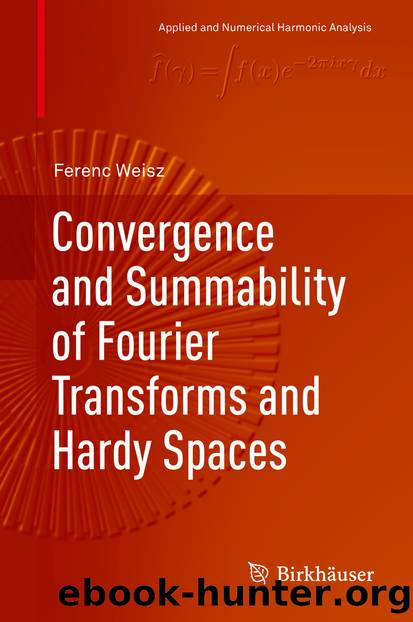﻿

# Convergence and Summability of Fourier Transforms and Hardy Spaces by Ferenc WeiszAuthor:Ferenc Weisz
Language: eng
Format: epub
Publisher: Springer International Publishing, Cham

and

where

and 1 < p < ∞. Thus Q 1 is a bounded projection on and so is Q = Q 1 P 1 + P 2 +. Since Qf satisfies (4.2.3), we obtain

Each function f can be rewritten as the sum of eight similar projections, which implies the theorem for q = ∞.

Equality (4.2.2) implies

which also shows the result for q = 1. ■

Theorem 4.2.8 does not hold for circular partial sums (see Stein and Weiss [311, p. 268] or Lu and Yan [239, p. 16]).

Theorem 4.2.9

If q = 2 and p < 2d∕(d + 1), then there exists a function whose circular partial sums s n q f diverge almost everywhere.